# Estimation of Kullback-Leibler losses

We address the question of estimating Kullback-Leibler losses rather than squared losses in recovery problems where the noise is distributed within the exponential family. We exhibit conditions under which these losses can be unbiasedly estimated or estimated with a controlled bias. Simulations on parameter selection problems in image denoising applications with Gamma and Poisson noises illustrate the interest of Kullback-Leibler losses and the proposed estimators.

Preprint available here

# Convergence of FISTA

Charles Dossal proved in 2015 with Antonin Chambolle the convergence of an extremely popular algorithm since its introduction in 2008 by Beck and Teboulle (for being extremely efficient in practice), the FISTA algorithm (Fast Iterative Shrinkage Algorithm). The convergence of the algorithm has remained an open problem in the communities of optimization/compressed sensing/image processing/machine learning for 7 years until Charles Dossal work.

# Scale-Space and Variational Methods

The team organized early June 2015 the international conference SSVM 2015 (Scale-Space and Variational Methods in Image Processing) in the Arcachon bay. More than 120 researchers from around the world participated. The conference proceedings were published in a book by Springer.

# Non parametric noise estimation

In order to provide a fully automatic denoising algorithm, we have developed an automatic noise estimation method that relies on the non-parametric detection of homogeneous areas. First, the homogeneous regions of the image are detected by computing Kendall’s rank correlation coefficient . Computed on neighboring pixel sequences, it indicates the dependancy between neighbors, hence reflects the presence of structure inside an image block. This test is non-parametric, so the performance of the detection is independant of the noise statistical distribution. Once the homogeneous areas are detected, the noise level function, i.e., the function of the noise variance with respect to the image intensities, is estimated as a second order polynomial minimizing theerror on the statistics of these regions.

Matlab implementation of the noise estimation algorithm

Related papers:

– C. Sutour, C.-A. Deledalle et J.-F. Aujol. Estimation of the noise level function based on a non-parametric detection of homogeneous image regions. Submitted to Siam Journal on Imaging Sciences, 2015.

– C. Sutour, C.-A. Deledalle et J.-F. Aujol. Estimation du niveau de bruit par la détection non paramétrique de zones homogènes. Submitted to Gretsi, 2015.

## References

 Buades, A., Coll, B., and Morel, J.-M. (2005). A review of image denoising algorithms, with a new one. Multiscale Modeling and Simulation, 4(2): 490–530.

The denoising algorithm that has been developed is based on an adaptive regularization of the NL-means . The proposed model is the following:

(1)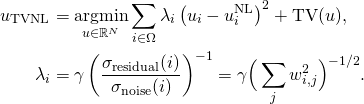where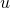is the solution obtained with the NL-means algorithm, TV refers to the total variation of the image and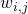is the weight that measures the similarity between the patch of indexand the patch of index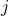in the NL-means algorithm. The ratio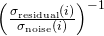reflects the noise variance reduction performed by the NL-means. This formulation allows locally adaptive regularization of the NL-means solution, thanks to a confidence index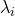that reflects the quality of the denoising performed by the NL-means.

This model can be adapted to the different noise statistics belonging to the exponential family (Gaussian, Poisson, multiplicative…). It can also be adapted to video denoising thanks to the use of 3D patches combined to a spatio-temporal TV regularization.

Matlab implementation of RNL

Results of video denoising with R-NL and comparisons

Related papers:
1. C. Sutour, C.-A. Deledalle et J.-F. Aujol. Adaptive regularization of the NL-means : Application to image and video denoising. IEEE Transactions on image processing, vol. 23(8) : 3506-3521, 2014.

2. C. Sutour, J.-F. Aujol, C.-A. Deledalle et J.-P. Domenger. Adaptive regularization of the NL-means for video denoising. International Conference on Image Processing (ICIP), pages 2704–2708. IEEE, 2014.

3. C. Sutour, J.-F. Aujol et C.-A. Deledalle. TV-NL : Une coopération entre les NL-means et les méthodes variationnelles. Gretsi, 2013.

## References

 Buades, A., Coll, B., and Morel, J.-M. (2005). A review of image denoising algorithms, with a new one. Multiscale Modeling and Simulation, 4(2): 490–530.

# Relaxations of non-convex fonctionals

We study different relaxations of non-convex functionals that can be found in image processing. Some problems, such as image segmentation, can indeed be written as the minimization of a functional. The minimizer of the functional represents the segmentation.

Different methods have been proposed in order to find local or global minima of the non-convex functional of the two-phase piecewise constant Mumford-Shah model. With a convex relaxation of this model we can find a global minimum of the non-convex functional. We present and compare some of these methods and we propose a new model with a narrow band. This models finds local minima while using robust convex optimization algorithms. Then a convex relaxation of a two-phase segmentation model is built that compares two given histograms with those of the two segmented regions.

We also study some relaxations of high-dimension multi-label problems such as optical flow computation. A convex relaxation with a new algorithm is proposed. The algorithm is iterative with exact projections. A new algorithm is given for a relaxation that is convex in each variable but that is not convex globally. We study the problem of constructing a solution of the original non-convex problem with a solution of the relaxed problem. We compare existing methods with new ones.

# Image colorization

Colorization is an important issue for example for the restoration of old documents, but also for the entertainment industry. The methods fall into two categories, manual methods and example-based methods for which the user provides a color image which serves as a source of information. The comparison of textures used to extract color. Regularization is then required, that is carried out in our case by minimizing a non-convex function. To propose a reasonable model for colorization and an efficient algorithm to minimize the proposed functional constituted the bulk of the beginning of the thesis.

However, methods-based example exhibited difficulty in finding a relevant source image. It is difficult to find a relevant source image for a given colorization, and the method fails frequently, just for example, when a color is not present in the source image, or when two smooth portions or similar textures expressed a different color. To avoid these issues, we proposed a method called collaborative colorization, based on an interactive method in which the user supervises the result. It has the ability to integrate color points in the result where the result appears unsatisfactory. This new information is integrated into the process by exploiting the non-convexity of the model.